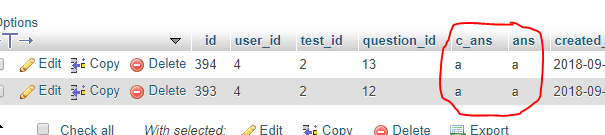# How to store JSON multiple array data database in Laravel?

I work on online exam portal after the complete exam I want to store result user detail on the database all data come from API.

Data look like

```{
"user_id": "420",
"test_id": "2",
"question_id": [
"12",
"13"
],
"c_ans": [
"a",
"b"
],
"ans": [
"a",
"b"
]```

}

My Question_id array data store successfully but not c_ans and an array.

My Controller

```   \$fbres       = new Testapp;
\$json        = \$request->all();
\$data        = \$json;

\$finalData = array();
\$blankArr = array();
//Check for array 1-d or 2-d
if(isset(\$data["user_id"])){
array_push(\$blankArr, \$data);
}else{
\$blankArr = \$data;
}

foreach (\$blankArr as \$key => \$value)
{

\$usr_id     = \$value['user_id']['0'];
\$test_id    = \$value['test_id']['0'];
\$c_ans      = \$value['c_ans']['0'];
\$ans        = \$value['ans']['0'];

foreach(\$value['question_id'] as \$k => \$v){
\$finalData = array('user_id'=> \$usr_id, 'question_id'=> \$v, 'test_id'=> \$test_id,'c_ans' => \$c_ans,'ans'=>\$ans);
\$store= \$fbres::insert(\$finalData);
}
}

if(\$store)
{
return response([
'Success' => "1",
'Message' => "Result Submitted Successfull..."
]);
}```

Asnwer storedContents

Thank you for visiting the Q&A section on Magenaut. Please note that all the answers may not help you solve the issue immediately. So please treat them as advisements. If you found the post helpful (or not), leave a comment & I’ll get back to you as soon as possible.

### Method 1

You can do something like this as per your data:

```\$fbres       = new Testapp;
\$json        = \$request->all();
\$data        = \$json;

\$finalData = array();
\$blankArr = array();
//Check for array 1-d or 2-d
if(isset(\$data["user_id"])){
array_push(\$blankArr, \$data);
}else{
\$blankArr = \$data;
}

foreach (\$blankArr as \$key => \$value)
{

\$usr_id     = \$value['user_id'];
\$test_id    = \$value['test_id'];

foreach(\$value['question_id'] as \$k => \$v){
\$c_ans      = \$value['c_ans'][\$k];
\$ans        = \$value['ans'][\$k];
\$finalData = array('user_id'=> \$usr_id, 'question_id'=> \$v, 'test_id'=> \$test_id,'c_ans' => \$c_ans,'ans'=>\$ans);
\$store= \$fbres::insert(\$finalData);
}
}

if(\$store)
{
return response([
'Success' => "1",
'Message' => "Result Submitted Successfull..."
]);
}```

Problem is \$c_ans, \$ans and quest_id have one to one mapping. So retrieve value also one by one.

All methods was sourced from stackoverflow.com or stackexchange.com, is licensed under cc by-sa 2.5, cc by-sa 3.0 and cc by-sa 4.0